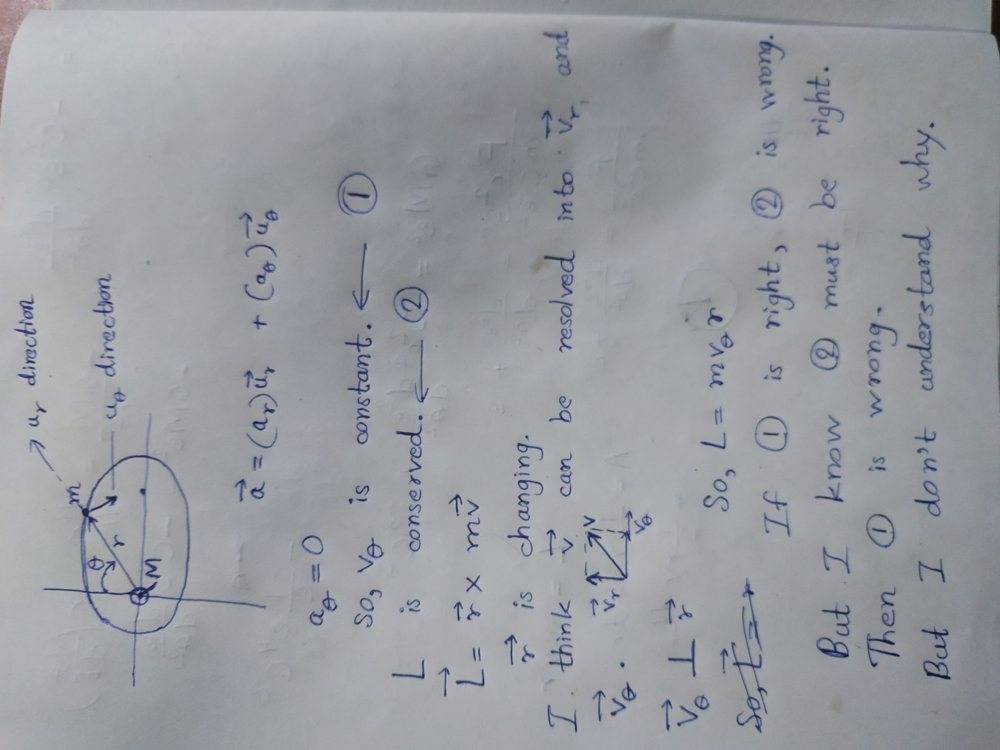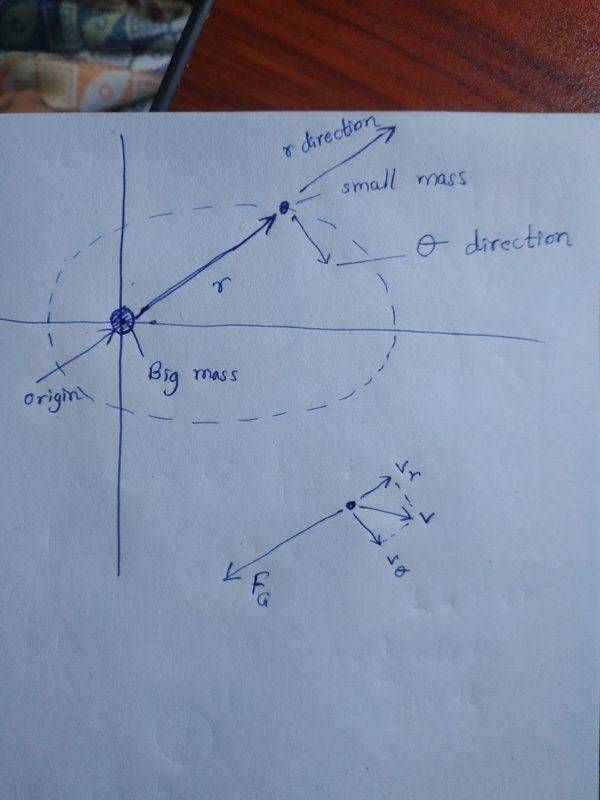# Elliptical orbit of planets

WaiYan
Homework Statement:
I'm confused about angular momentum conservation and the polar component velocities.
Relevant Equations:
I know that angular momentum is conserved in planetary motion.When I think the motion in terms of polar coordinate system, theta component of velocity seems to be constant.But I think if theta component of velocity is constant, angular momentum is not conserved.So, theta component must change somehow.But I can't think why that component of velocity changes.Cutter Ketch
Yes, the angular velocity changes. I am guessing your question is something like, if the force is always “toward the center”, why does the angular velocity change? Well, “toward the center” is the wrong way to think about it. That only makes sense for circular orbits. Consider an elliptical orbit. There are two places where the force is perpendicular to the velocity vector. Consider the apogee. Yes the force is perpendicular to the path and does not cause angular acceleration. However, the speed is too slow. The force is greater than is required to keep moving in a circle. The path deviates from a circle, and so the force is no longer perpendicular to the velocity vector. Now the force is partially pulling in the direction of motion and the speed increases as does the angular velocity.

in short, if the speed is wrong for a circle you don’t get a circle, and if it isn’t a circle then the force isn’t always perpendicular to the motion.

•WaiYan
WaiYan
I don't think the force is acting towards centre.But I think the force is always towards the big mass M.Right?When I think this situation in terms of polar coordinates, I put the big mass at the origin of coordinate system(But for ellipse, at one focus).In polar coordinates, I can resolve the velocity into the r component and theta component.R direction and theta direction are always perpendicular.Force is always along r direction and so there is no acceleration in theta direction.So component of v in theta direction must not change in magnitude.I mean that.Is that correct?WaiYan
Yes, the angular velocity changes. I am guessing your question is something like, if the force is always “toward the center”, why does the angular velocity change? Well, “toward the center” is the wrong way to think about it. That only makes sense for circular orbits. Consider an elliptical orbit. There are two places where the force is perpendicular to the velocity vector. Consider the apogee. Yes the force is perpendicular to the path and does not cause angular acceleration. However, the speed is too slow. The force is greater than is required to keep moving in a circle. The path deviates from a circle, and so the force is no longer perpendicular to the velocity vector. Now the force is partially pulling in the direction of motion and the speed increases as does the angular velocity.

in short, if the speed is wrong for a circle you don’t get a circle, and if it isn’t a circle then the force isn’t always perpendicular to the motion.
I don't think the force is acting towards centre.But I think the force is always towards the big mass M.Right?When I think this situation in terms of polar coordinates, I put the big mass at the origin of coordinate system(But for ellipse, at one focus).In polar coordinates, I can resolve the velocity into the r component and theta component.R direction and theta direction are always perpendicular.Force is always along r direction and so there is no acceleration in theta direction.So component of v in theta direction must not change in magnitude.I mean that.Is that correct?

#### Attachments

Homework Helper
Force is always along r direction and so there is no acceleration in theta direction.So component of v in theta direction must not change in magnitude.I mean that.Is that correct?
No, that is not correct.

The problem is that as the particle's angle changes, the component of velocity in the radial direction is being traded for a component of velocity in the tangential direction. An incremental clockwise change in theta turns a bit of inward radial velocity into a bit of clockwise tangential velocity. And it turns a bit of tangential velocity into a bit of outward radial velocity.

A similar way of thinking about it is that by considering component-wise acceleration relative to the current rotation rate, you have implicitly adopted a rotating frame and are neglecting the Coriolis force.

•WaiYan
WaiYan
No, that is not correct.

The problem is that as the particle's angle changes, the component of velocity in the radial direction is being traded for a component of velocity in the tangential direction. An incremental clockwise change in theta turns a bit of inward radial velocity into a bit of clockwise tangential velocity. And it turns a bit of tangential velocity into a bit of outward radial velocity.

A similar way of thinking about it is that by considering component-wise acceleration relative to the current rotation rate, you have implicitly adopted a rotating frame and are neglecting the Coriolis force.